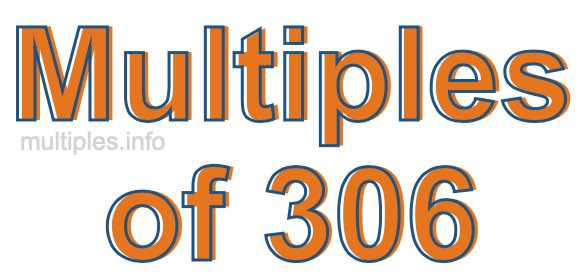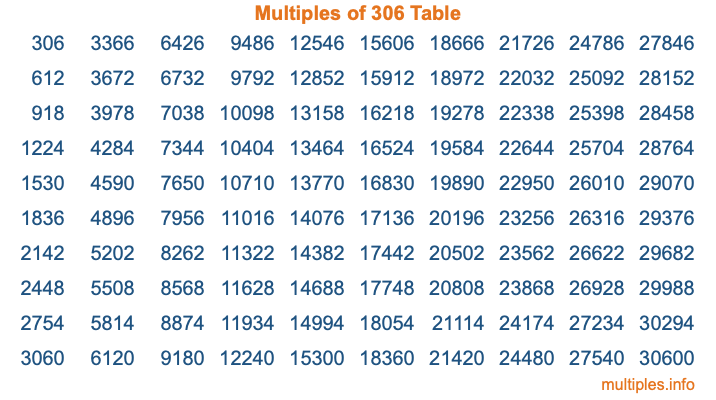Multiples of 306Welcome to the Multiples of 306 page. Here we will first teach you everything you will ever need to know about the multiples of 306, and then give you a study guide summary of everything we taught you to make sure you remember it all. Use this page to look up facts and learn information about the multiples of 306. This page will make you a multiples of three hundred six expert!

Definition of Multiples of 306
Multiples of 306 are all the numbers that when divided by 306 equal an integer. Each of the multiples of 306 are called a multiple. A multiple of 306 is created by multiplying 306 by an integer.

Therefore, to create a list of multiples of 306, you start with 1 multiplied by 306, then 2 multiplied by 306, then 3 multiplied by 306, and so on for as long as you want. Thus, the list of the first five multiples of 306 is 306, 612, 918, 1224, and 1530. To see a larger list of multiples of 306, see the printable image of Multiples of 306 further down on this page. We also have a category where you can choose any nth multiple of 306.

Multiples of 306 Checker
The Multiples of 306 Checker below checks to see if any number of your choice is a multiple of 306. In other words, it checks to see if there is any number (integer) that when multiplied by 306 will equal your number. To do that, we divide your number by 306. If the the quotient is an integer, then your number is a multiple of 306.

Is  a multiple of 306?

Least Common Multiple of 306 and ...
A Least Common Multiple (LCM) is the lowest multiple that two or more numbers have in common. This is also called the smallest common multiple or lowest common multiple and is useful to know when you are adding our subtracting fractions. Enter one or more numbers below (306 is already entered) to find the LCM.

Check out our LCM Calculator if you need more details about the Least Common Multiple or if you need the LCM for different numbers for adding and subtraction fractions.

nth Multiple of 306
As we stated above, 306 is the first multiple of 306, 612 is the second multiple of 306, 918 is the third multiple of 306, and so on. Enter a number below to find the nth multiple of 306.

th multiple of 306

Multiples of 306 vs Factors of 306
306 is a multiple of 306 and a factor of 306, but that is where the similarities end. All postive multiples of 306 are 306 or greater than 306. All positive factors of 306 are 306 or less than 306.

Below is the beginning list of multiples of 306 and the factors of 306 so you can compare:

Multiples of 306: 306, 612, 918, 1224, 1530, etc.

Factors of 306: 1, 2, 3, 6, 9, 17, 18, 34, 51, 102, 153, 306

As you can see, the multiples of 306 are all the numbers that you can divide by 306 to get a whole number. The factors of 306, on the other hand, are all the whole numbers that you can multiply by another whole number to get 306.

It's also interesting to note that if a number (x) is a factor of 306, then 306 will also be a multiple of that number (x).

Multiples of 306 vs Divisors of 306
The divisors of 306 are all the integers that 306 can be divided by evenly. Below is a list of the divisors of 306.

Divisors of 306: 1, 2, 3, 6, 9, 17, 18, 34, 51, 102, 153, 306

The interesting thing to note here is that if you take any multiple of 306 and divide it by a divisor of 306, you will see that the quotient is an integer.

Multiples of 306 Table
Below is an image of the first 100 multiples of 306 in a table. The table is in chronological order, column by column. The first column has the first ten multiples of 306, the second column has the next ten multiples of 306, and so on.The Multiples of 306 Table is also referred to as the 306 Times Table or Times Table of 306. You are welcome to print out our table for your studies.

Negative Multiples of 306
Although not often discussed or needed in math, it is worth mentioning that you can make a list of negative multiples of 306 by multiplying 306 by -1, then by -2, then by -3, and so on, to get the following list of negative multiples of 306:

-306, -612, -918, -1224, -1530, etc.

Multiples of 306 Summary
Below is a summary of important Multiples of 306 facts that we have discussed on this page. To retain the knowledge on this page, we recommend that you read through the summary and explain to yourself or a study partner why they hold true.

There are an infinite number of multiples of 306.

A multiple of 306 divided by 306 will equal a whole number.

306 divided by a factor of 306 equals a divisor of 306.

The nth multiple of 306 is n times 306.

The largest factor of 306 is equal to the first positive multiple of 306.

306 is a multiple of every factor of 306.

306 is a multiple of 306.

A multiple of 306 divided by a divisor of 306 equals an integer.

306 divided by a divisor of 306 equals a factor of 306.

Any integer times 306 will equal a multiple of 306.

Multiples of a Number
Here you can get the multiples of another number, all with the same attention to detail as we did for multiples of 306 on this page.

Multiples of
Multiples of 307
Did you find our page about multiples of three hundred six educational? Do you want more knowledge? Check out the multiples of the next number on our list!

Copyright  |   Privacy Policy  |   Disclaimer  |   Contact